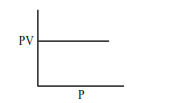# Which one of the following is the correct PV vs P plotQuestion:

Which one of the following is the correct PV vs P plot at constant temperature for an ideal gas ? (P and $\mathrm{V}$ stand for pressure and volume of the gas respectively)

1.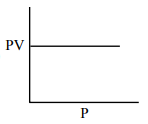2.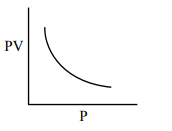3.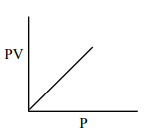4.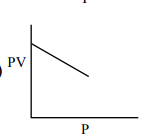Correct Option: 1

Solution:

$\mathrm{PV}=\mathrm{nRT}(\mathrm{n}, \mathrm{T}$ constant $)$

$\mathrm{PV}=$ constant